Physics - Classical Mechanics - Explaining the Physics behind Satellite Motion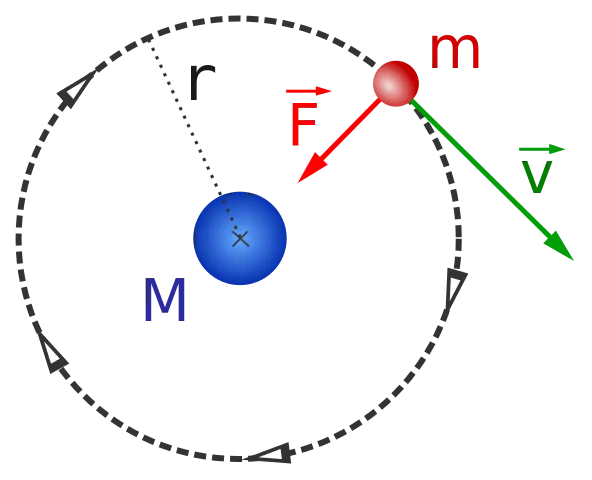[Image1]

Introduction

Hey it's a me again @drifter1!

In this article we will continue with Physics, and more specifically the branch of "Classical Mechanics". Today's article is about Satellite Motion that fits right into the chapter of Gravity.

So, without further ado, let's get straight into it!

Types of Satellites

Satellites are split into two categories: natural satellites and man-made satellites. The moon, planets and coments are natural satellites, whilst man-made satellites are launched into orbit for purposes of telecommunication, scientific research, weather forecasting, intelligence etc. Either way, both types are following the same base physics principles and described by the same math.

Why Satellites don't fall back to Earth?

To understand satellites one has to think of them as projectiles that are only affected by the force of gravity. Once launched into orbit, or while in orbit, the only force that affects their motion is the force of gravity. When the speed of the satellite is sufficient enough then the satellite can orbit around a massive object, like the Earth for example. The motion of the satellite is then circular, or elliptical to be more exact. If the speed is too small it eventually falls back to the Earth. The same also happens if it's velocity is "too parallel" to the gravity force. For the best results the velocity should be perpendicular to the force of gravity.

So, to summarize, its possible to launch a man-made object into space, and hitting the correct criteria of velocity, the then called satellite would start orbiting the Earth, without falling.

The Circular Motion of Satellites

The motion of a satellite can be described as circular as:

• The velocity of the satellite is tangent to the circular path it moves along (and perpendicular to the force of gravity)
• The force of gravity is directed inwards, towards the center of the circular path (or body it orbits)
• The acceleration caused by the force of gravity also points in the same direction
Thus, in the case of satellite motion, the force of gravity plays the role of the centripetal force. If no gravity force was present then the satellite would continue moving at the same speed and in the same direction, as Newton's First Law of motion states.

The Relationship of Radius and Velocity

From the physics of circular motion the perpedincular to the motion centripetal forcec is given by the equation: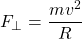• F: centripetal force
• m: mass of the satellite
• v: velocity of the circular motion
• R: radius of the circular motion
The force of gravity is given by the equation: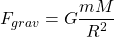• Fgrav: force of gravity
• G: Gravitational Constant (6.674 × 10-11 N)
• m: mass of the satellite
• M: mass of the central body
• R: distance between the two bodies
Since the force of gravity is playing the role of the centripetal force, F = Fgrav giving:This final equation shows us that the velocity and radius of the satellite's motion are related to each other:
• The closer (R ↓) a satellite is to the central body, the faster it moves (velocity ↑)
• The further away (R ↑) a satellite is to the central body, the slower it moves (velocity ↓)

Acceleration of the Satellite

In previous articles, the acceleration of gravity was proved to be: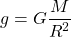This is the acceleration of the circular motion of the satellite.

Orbital Period

Lastly, an circular motion is also defined by a period T. From circular motion, its known that the period can be calculated using: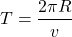Substituting the equation of velocity v into this equation, :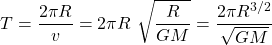Its also common to square the whole equation to get: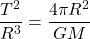Either way, the period is larger (T ↑) when the radius is larger (R ↑) or the velocity is smaller (v ↓).

Its also worth noting that no equation contains the mass of the satellite, because velocity, acceleration and period are only dependent upon the radius of the orbit and the mass of the central body.

RESOURCES:

Images

1.Final words | Next up

And this is actually it for today's post!

Next time we will get into Kepler's Laws of Planetary Motion...

See ya!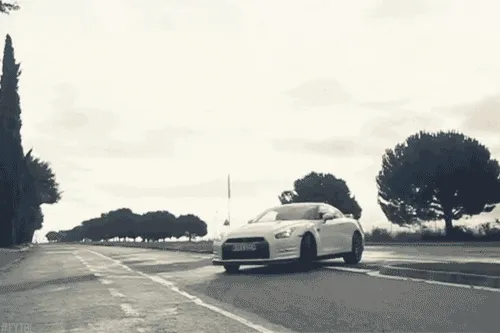Keep on drifting!
H2
H3
H4
3 columns
2 columns
1 column
1 Comment